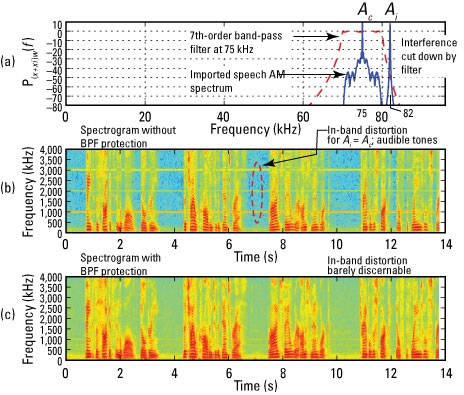##### Signals and Systems For DummiesYou can test the AM receiver by using an actual voice message. The value of this test lies in actually listening to the speech before and after it goes through the communications link. Your ears hear differences that aren’t always easy to discern from graphical displays alone.

Also, because the voice message occupies a wider spectral bandwidth than the single sinusoid, the nonlinear signal processing of the envelope detector is more realistically characterized in the presence of strong interference.

You can access the full code listing for an end-to-end simulation in the Python module ssd.py. The simulation employs multirate digital signal processing (DSP) so that a real voice message, sampled at 8 ksps, can be processed through the AM transceiver.

The message signal at 8 ksps is upsampled by 24 to 192 ksps. In this simulation, a carrier frequency of 75 kHz (can be viewed as an emulation of the 455 kHz IF) is used with the interference signal at 82 kHz (7 kHz above the carrier). The envelope detector is an ideal halfwave rectifier (in software) followed by a low-pass filter and a level shifter (subtract signal mean).

The signal is then downsampled by 24 to exit the receiver at 8 ksps. Listening tests of the speech text vector confirm the elimination of in-band tones when a seventh-order Chebyshev BPF is placed at the input to the envelope detector.

See the simulation results in the following figure.Credit: Illustration by Mark Wickert, PhD

Here are the abbreviated IPython commands:

```In [<b>964</b>]: fs, m_wav = ssd.from_wav('OSR_us_000_0030_8k.wav')
In [<b>965</b>]: m_wav = m_wav[10000:120000] #truncate the speech
In [<b>967</b>]: x192w, t192w, m24 = ssd.am_tx(m_wav,0.8)# tx sig
In [<b>968</b>]: xi192w = cos(2*pi*82.0e3*t192w)# interference
In [<b>969</b>]: m_rx8,t8,m_rx192,x_env_det = ssd.am_rx(x192w+xi192w) # form rx signal at 8k & 192k, no BPF however
In [<b>973</b>]: Pxx1,f = psd(x192w+xi192w,2**12,192)
In [<b>984</b>]: specgram(m_rx8,2**8,8000); # plot spectrogram
In [<b>987</b>]: x192fw = signal.lfilter(b_bpf,a_bpf,x192w+xi192w)# BPF
In [<b>988</b>]: m_rx8,t8,m_rx192,x_env_det = ssd.am_rx(x192fw)
In [<b>995</b>]: specgram(m_rx8,2**8,8000); # plot spectrogram
In [<b>996</b>]: ssd.to_wav('speech_test.wav',8000,m_rx8)# listen```

In Figure a, the AM signal appears as a symmetrical blob with a carrier spike poking up the middle, right at 75 kHz. The interference is located at 82 kHz, unfiltered at present.

The BPF filter shape is superimposed in the plot of Figure a so you can see how the interference is cut down. Figures b and c show you the impact of the BPF. The spectrogram plot is used here, which displays the frequency spectrum versus time.

The interference effectively sits outside the 5-kHz audio band-pass, but due to the nonlinear signal processing action of the envelope detector, in-band tones at 1, 3, and 3 kHz are evident in the spectrogram of Figure b. With the BPF in place, the tones go away (Figure c).